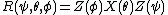# Euler Angles

Also found in: Wikipedia.

## Euler angles

Three angular parameters that specify the orientation of a body with respect to reference axes. They are used for describing rotating systems such as gyroscopes, tops, molecules, and nonspherical nuclei. They are not symmetrical in the three angles but are simpler to use than other rotational parameters.

Unfortunately, different definitions of Euler's angles are used, and therefore it is confusing to compare equations in different references. The definition given here is the majority convention according to H. Margenau and G. Murphy.

Let OXYZ be a right-handed cartesian (right-angled) set of fixed coordinate axes and Oxyz a set attached to the rotating body (see illustration).

The orientation of Oxyz can be produced by three successive rotations about the fixed axes starting with Oxyz parallel to OXYZ. Rotate through (1) the angle ψ counterclockwise about OZ, (2) the angle Θ counterclockwise about OX, and (3) the angle &phgr; counterclockwise about OZ again. The line of intersection OK of the xy and XY planes is called the line of nodes.

Denote a rotation about OZ, for example, by Z (angle). Then the complete rotation is, symbolically, given by the equation below where the rightmost operation is donefirst.

McGraw-Hill Concise Encyclopedia of Physics. © 2002 by The McGraw-Hill Companies, Inc.
The following article is from The Great Soviet Encyclopedia (1979). It might be outdated or ideologically biased.

## Euler Angles

(or Eulerian angles), the angles φ, θ, and ψ that define the position of a rectangular Cartesian coordinate system OXYZ with respect to another rectangular Cartesian coordinate system Oxyz of the same orientation (see Figure 1).Figure 1

Let OK be an axis, called the line of nodes, that coincides with the line of intersection of the coordinate plane Oxy of the first system with the coordinate plane OXY of the second system and is directed so that the axes Oz, OZ, and OK form a trihedral of the same orientation. The Euler angle φ, or angle of spin, is the angle between the axes Ox and OK, which is measured in the plane Oxy from Ox in the direction of the shortest rotation from Ox to Oy. The Euler angle θ, or angle of nutation, is the angle between Oz and OZ; it does not exceed π. The Euler angle ψ, or angle of precession, is the angle between the axes OK and OX, which is measured in the plane OXY from Ok in the direction of the shortest rotation from OX to OY. When θ = 0 or π, the Euler angles are not defined.

The Euler angles were introduced by L. Euler in 1748 and are used extensively in the dynamics of solids—for example, in the theory of the gyroscope—and in celestial mechanics.

## Euler angles

[′ȯi·lər ‚aŋ·gəlz]
(mechanics)
Three angular parameters that specify the orientation of a body with respect to reference axes.
McGraw-Hill Dictionary of Scientific & Technical Terms, 6E, Copyright © 2003 by The McGraw-Hill Companies, Inc.
References in periodicals archive ?
By comparison to (3) and solving the three (overdetermined) equations for each leg, the input angle [[theta].sub.i] and Euler angles [[phi].sub.x] and [[phi].sub.y] can be calculated to describe the singular configuration.
In the inverse geometric problem however, it can be considered that the three Euler angles [[alpha].sub.1], [[alpha].sub.2], [[alpha].sub.3] give the absolute orientation of the mobile platform.
OVCs are designed with output variance constraints on helicopter Euler angles (i.e., [10.sup.-4]), while all four helicopter controls are used as inputs.
In this section, the navigation board and the algorithm for the computation of the Euler angles are described.
Euler angles [psi], [theta], and [phi] describe an orientation of frame B achieved by the sequential rotations, from alignment with frame A, of [psi] around [z.sub.A], [theta] around [y.sub.A], and [phi] around [x.sub.A].
The rotation matrix A is chosen randomly by the Euler angles: yaw, pitch, and roll within (-90, 90) x (-90, 90] x [0, 360) degree.
In Euler's theorem, an attitude can be represented by a single rotation angle about a principal axis, called the eigen-axis, or three sequential rotation angles, called the Euler angles. A rest-to-rest maneuver to a target attitude can then be performed by either an eigen-axis maneuver or three sequential principal submaneuvers following Euler angle sequences.
As is known, the orientation parameters consist of the roll, pitch, and yaw angles, namely, the frequently used Euler angles .
Hence, we set that the capture coordinate system coincides with the camera coordinate system [O.sub.C][X.sub.C][Y.sub.C][Z.sub.C], and assume the Euler angles between capture coordinate system [O.sub.C][X.sub.C][Y.sub.C][Z.sub.C] and target coordinate system [O.sub.Z][X.sub.Z][Y.sub.Z][Z.sub.Z] as [alpha], [beta], [gamma], respectively .
In , a linear system error model based on the Euler angles errors expressing the local frame errors is developed, and the corresponding system observation model is derived.
This issue is related to the positions of the platforms, Euler angles of the platforms (yaw, pitch, and roll), and antenna gains .
Table 1: Euler angles and Burgers orientation relationship of all 12 variants.

Site: Follow: Share:
Open / Close